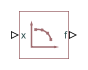# PS Lookup Table (1D)

Approximate one-dimensional function using specified lookup method

•Libraries:
Simscape / Foundation Library / Physical Signals / Lookup Tables

## Description

The PS Lookup Table (1D) block computes an approximation to some function `f=f(x)` given data vectors `x` and `f`. Both the input and the output are physical signals.

The length of the `x` and `f` data vectors provided to this block must match. Also, the `x` data vector must be strictly monotonic, either increasing or decreasing.

You define the lookup table by specifying the Table grid vector parameter as a 1-by-`n` vector (`x` data vector) and the Table values parameter as a 1-by-`n` vector (`f` data vector). The block generates output based on the input values using the selected interpolation and extrapolation methods. You have a choice of two interpolation methods and two extrapolation methods. You also have an option for the block to issue an error if the input signal value is outside the table grid vector range.

### Plotting Table Data

Plotting a lookup table lets you visualize the data before simulating the model, to make sure that the table is correct. The plot reflects tabulated data specified for the block, as well as the selected interpolation and extrapolation options.

To plot the data, right-click the block in your model and, from the context menu, select Foundation Library > Plot Table. For more information, see Plot Lookup Tables.

## Ports

### Input

expand all

Input query point, or a row or column vector of query points, along the x-axis. The signal size is either a scalar (for a single query point), or a row or column vector representing the coordinates of the query points along the x-axis.

### Output

expand all

Output function value, based on applying the lookup table to the input value. The output signal size matches the input signal size. The output signal unit is determined by the unit of the Table values parameter.

## Parameters

expand all

Specify the vector of input values as a one-dimensional array. The input values vector must be strictly monotonic, either increasing or decreasing. The values can be nonuniformly spaced. For smooth interpolation, the vector must contain at least three values. For linear interpolation, two values are sufficient.

Parameter unit must be commensurate with the unit of the input signal at port x. By default, the unit is `1` (unitless). You can select a different unit from the drop-down list or type the desired unit name, such as `rpm`, or a valid expression, such as `rad/s`. For more information and a list of unit abbreviations, see How to Specify Units in Block Dialogs and Unit Definitions.

Specify the vector of output values as a one-dimensional array. The output values vector must be of the same size as the input values vector.

Parameter unit determines the unit of the output signal at port f. By default, the unit is `1` (unitless). You can select a different unit from the drop-down list or type the desired unit name, such as `rpm`, or a valid expression, such as `rad/s`. For more information and a list of unit abbreviations, see How to Specify Units in Block Dialogs and Unit Definitions.

Select one of the following interpolation methods for approximating the output value when the input value is between two consecutive grid points:

• `Linear` — Uses a linear function. Select this option to get the best performance.

• `Smooth` — Uses a modified Akima interpolation algorithm. For details, see `tablelookup`. Select this option to produce a continuous curve with continuous first-order derivatives.

Select one of the following extrapolation methods for determining the output value when the input value is outside the range specified in the argument list:

• `Linear` — Extends from the edge of the interpolation region linearly. The slope of the linear extrapolation is equal to the slope of the interpolated curve at the edge of the interpolation region.

• `Nearest` — Extends from the edge of the interpolation region as a constant. The value of the nearest extrapolation is equal to the value of the interpolated curve at the edge of the interpolation region. Select this option to produce an extrapolation that does not go above the highest point in the data or below the lowest point in the data.

• `Error` — Issues an error if the input signal is outside the range of the table. Select this option to avoid going into the extrapolation mode when you want your data to be within the table range.

## Version History

Introduced in R2007a

expand all